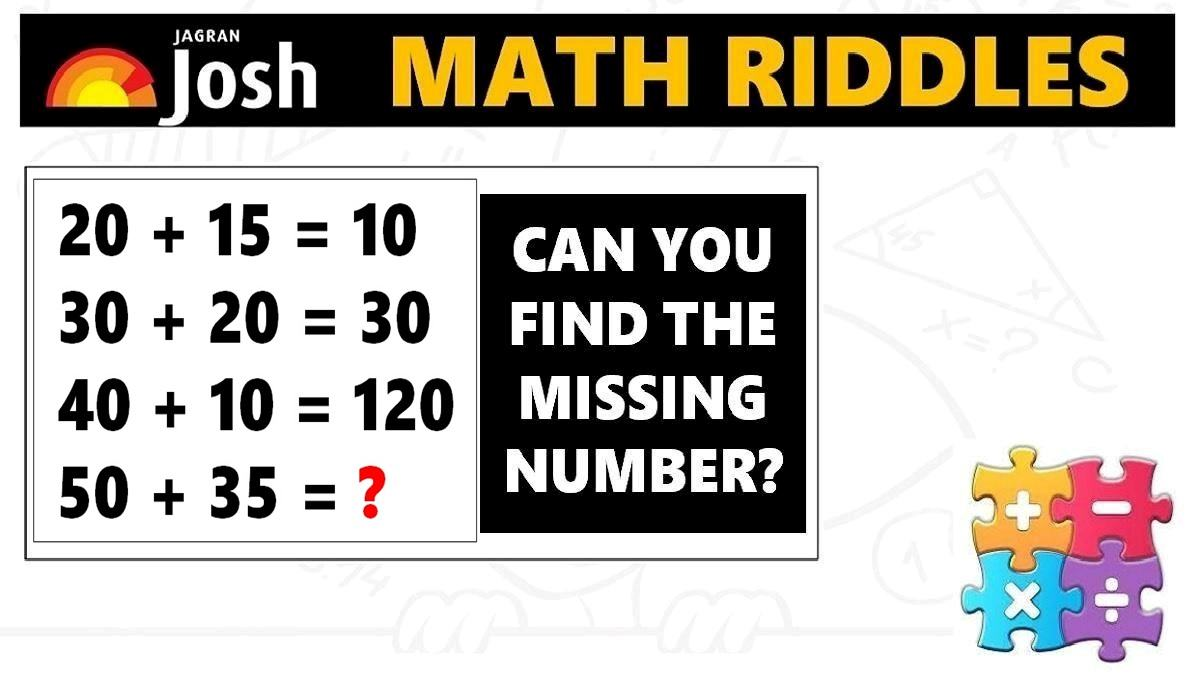# Math Riddles with Answers: 95% People Could Not Solve This Math Problem, Can You?

Math Riddles Challenge: This math puzzle will trick your brain. Check your IQ Levels with this Mathematics Puzzle.Math Riddles with Answers: 95% People Could Not Solve This Math Problem, Can You?

Math Riddles: Can you solve this tricky mathematics puzzle in less than 1 minute? Do you see the logic in it? Well, 95% people could not solve this math riddle. We challenge you to find the missing number in this math riddle in less than 1 minute. This mathematics puzzle will surely a great brain exercise. You will love this math riddle, after all maths is fun, isn’t it? Let us begin.

Explanation:

## Tell us in comments: Did you solve this mathematics puzzle in less than 1 minute?

Check out more math puzzles!